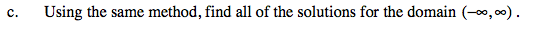### Home > PC > Chapter 6 > Lesson 6.1.3 > Problem6-41

6-41.
1. Many times when we are solving trigonometric equations, the solutions are not convenient values on the unit circle where we can find the exact answers. For example, when we solve 2 sin x = 1, we get x = sin−1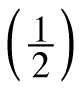and we know the exact solution between −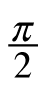andis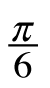. The other solution between 0 and 2π is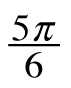. But what happens when we try to solve 5 sin x = 4? Homework Help ✎

1. Solve 5 sin x = 4 for x and calculate a decimal approximation.

2. Is this the only solution between 0 and 2π? Use what you know about the symmetry of the sine wave to determine the other solution between 0 and 2π to this trigonometric equation.

3. Using the same method, find all of the solutions for the domain (−∞, ∞).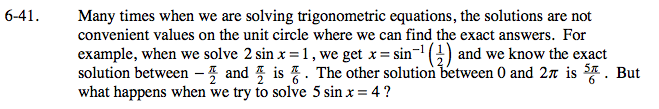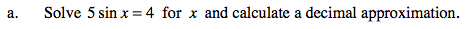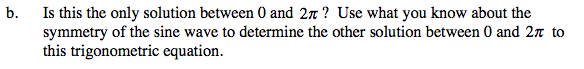Use the unit circle and symmetry.

$\text{Where else is the sin }x=\frac{4}{5}?$

How can you write this?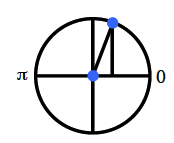x = 0.927
x = π − 0.927